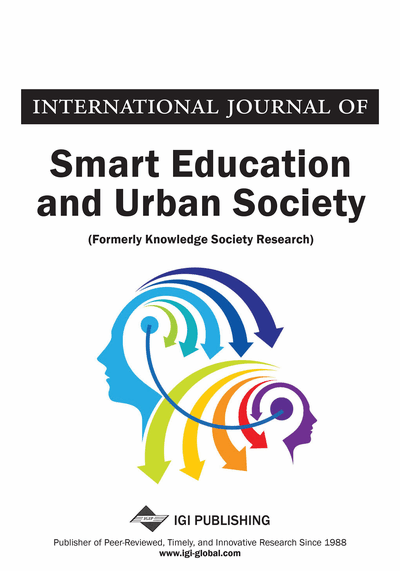# Tu-vera: An Encryption Algorithm Using Propositional Logic Calculus

Maria Vargas-Vera
DOI: 10.4018/IJSEUS.2018040105
OnDemand:
(Individual Articles)
Available
\$29.50
No Current Special Offers

## Abstract

This article describes how the encryption algorithm (called Tu-vera) depends on the transformation of a phrase written in English into a sequence of propositional logic formulas which can be understand by a human receiver. This happens if the receiver has a set of reserved words and he/she knows the level of unfolding manipulation that the receiver needs to perform in the transformation of the phrase/sentence. The Tu-vera algorithm requires several steps like a) to give a phrase; b) to re-order words of the given phrase in order to form a propositional logic formula; c) to make use of background knowledge by performing substitutions; d) to answer questions in general subjects (like literature, biology and so forth); e) to change synonyms/antonyms (if this is feasible); f) to perform actions in order to give value to both or one operand of the logic formula and g) to conclude the final answer of the logic formula (true or false) depending of the logic values of the operands in the logic formula. Finally, a working example, in the subject of universal history is introduced.
Article Preview
Top

The section of related work is organised in two streams namely, a section of first order logic calculus and a section of encryption algorithms.

### Mathematical Logic

• Definition 1: A Model is a set D and a function f such that:

• The function f assigns each constant to a member of D.

• The function f assigns each unary predicate to a subset of D.

• The function f assigns each binary predicate to a subset D × D.

The basic idea is that a set of constants and predicates are paired with elements from the set of elements of the model. In other words, each constant can be paired directly with an element of the model. For, example, if our model includes an individual called John, then, we might pair the constant “a” with the individual John.

Let us consider predicates. An expression like G (a) is true just in case f (a) is in the subset of D that f assigns G to. For example, if “a” is paired with “John” and John is in the set of D that G is paired with, f (a) = John and Johnf(G), then G(a) is true.

In the same way, H (a, b) is true just in case “(a, b)” is in the subset of D × D that f assigns H to. For example, if we take D to be the set of words of English and we take H to be the relation has fewer letters than, then H (a, b) is true just in case the elements we pair constant “a” and constant “b” which are in the set of ordered pairs defined by f (H). For example, if f (a) = table and f (b) = vase, then (table, vase) ∈ f (H) and H (a, b) is true.

Note that there is not requirement that there be a single model. AQ logical system can be paired with any number of models. A more detailed description can be found in (Mendelson, 2009).

## Complete Article List

Search this Journal:
Reset
Volume 14: 1 Issue (2023): Forthcoming, Available for Pre-Order
Volume 13: 4 Issues (2022): 1 Released, 3 Forthcoming
Volume 12: 4 Issues (2021)
Volume 11: 4 Issues (2020)
Volume 10: 4 Issues (2019)
Volume 9: 4 Issues (2018)
View Complete Journal Contents Listing